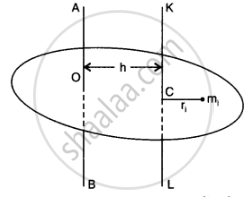CBSE (Science) Class 11CBSE
Share

# Prove the Theorem of Parallel Axes If the Centre of Mass is Chosen to Be the Origin Sum Miri=0 - CBSE (Science) Class 11 - Physics

ConceptTheorems of Perpendicular and Parallel Axes

#### Question

Prove the theorem of parallel axes.

(Hint: If the centre of mass is chosen to be the origin summ_ir_i = 0

#### Solution

Theorem of parallel axes: According to this theorem, moment of inertia of a rigid body about any axis AB is equal to moment of inertia of the body about another axis KL passing through centre of mass C of the body in a direction parallel to AB, plus the product of total mass M of the body and square of the perpendicular distance between the two parallel axes. If h is perpendicular distance between the axes AB and KL, then Suppose the rigid body is made up of n particles m1, m2, …. mn, mn at perpendicular distances r1, r2, ri…. rn. respectively from the axis KL passing through the centre of mass C of the body.

If h is the perpendicular distance of the particle of mass m{ from KL, thenThe perpendicular distance of ith particular from the axis

AB = (r_i + n)

or I_(AB) = sum_(i)m_i(r_i + h)^2

= sum_(i) m_ir_i^2 + sum_(i) m_ih^2 + 2h sum_(i) m_ir_i ....(ii)

As the body is balanced about the centre of mass the algebraic sum of the moments of the weights of all particles about an axis passing through C must be zero

sum_(i)(m_ig)r_i = 0 or g sum_(i) m_ir_i or sum_(i) m_ir_i = 0  ...(iii)

From equation (ii) we have

I_(AB) = sum_(i) m_ir_i^2 + (summ_i)h^2 + 0

or I_(AB) = I_(KL) + Mh^2

Where I_"KL" = sum_(i) m_ir_i^2  and M = sum m_i

Is there an error in this question or solution?

#### APPEARS IN

NCERT Solution for Physics Textbook for Class 11 (2018 to Current)
Chapter 7: System of Particles and Rotational Motion
Q: 26.2 | Page no. 180

#### Video TutorialsVIEW ALL 

Solution Prove the Theorem of Parallel Axes If the Centre of Mass is Chosen to Be the Origin Sum Miri=0 Concept: Theorems of Perpendicular and Parallel Axes.
S